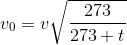Resonance Column

# Materials Required

• Resonance column apparatus
• Tuning forks of known frequency
• Rubber hammer

# Real Lab Procedure

• The inner tube of the resonance column is lowered to minimum so that the length of air column inside the tube is very small.
• Take a tuning fork of known frequency and striking it with a rubber hammer. The tuning fork now produces longitudinal waves with a frequency equal to the natural frequency of the tuning fork.
• Place the vibrating tuning fork horizontally above the tube. Sound waves are passes down to the tube and reflect back at the water surface.
• Now, raise the tube and the tuning fork until a maximum sound is heard.
• Measure the length of air column at that position. This is taken as the first resonant length, l1.
• Then raise the tube approximately about three times the first resonant length.
• Excite the tuning fork again and place it on the mouth of the tube.
• Change the height of the tube until the maximum sound is heard.
• Measure the length of air column at that position. This is taken as the second resonant length, l2.
• We can now calculate the velocity of sound in air at room temperature by using the relation,• Repeat the experiment with tuning forks of different frequencies and in each time, calculate the value of v.
• The mean of the calculated values will give the velocity of sound in air at room temperature.
• By using the value of v, we can calculate the velocity of sound in air at 00C as,Where,  v0 - velocity of sound in air at 00C.

v  - velocity of sound in air at temperature t.

t  - Room temperature.

# Simulator Procedure (as performed through the Online Labs)

• Select the surrounding from the drop down list.
• Select the surrounding temperature using the slider.
• Select the diameter of the tube using the slider.
• Select the frequency of the tuning fork using the slider.
• Click on the ‘Hit tuning fork’ button to start/stop vibration.
• Click on the ‘Sound On’ button to hear the sound.
• Drag the inner tube/Change the slider to adjust the height of the air column
• Drag the apparatus to move it down.
• Click on the zoom in icon to view the zoomed area.
• Click on the ‘Show result’ button to see the result.
• To redo the experiment, click on the ‘Reset’ button.

# Observations

 No. Frequency of tuning fork, ν (Hz) First resonating length, l1 (cm) Second resonating length, l2 (cm) l2-l1 (×10-2 cm) Velocity,(m/s) 1 2 Mean 1 2 Mean 1 2 3 4 5

# Calculations

Room temperature, t                                      = ............. 0C

Velocity of sound in air at room temperature, v = ………... m/s

Velocity of sound in air at 00C,= ............. m/s

# Result

Velocity of sound in air at room temperature, v =……....... m/s

Cite this Simulator: